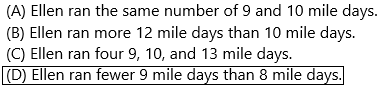# Texas Go Math Grade 4 Lesson 17.1 Answer Key Frequency Tables

Refer to our Texas Go Math Grade 4 Answer Key Pdf to score good marks in the exams. Test yourself by practising the problems from Texas Go Math Grade 4 Lesson 17.1 Answer Key Frequency Tables.

## Texas Go Math Grade 4 Lesson 17.1 Answer Key Frequency Tables

Essential Question

How do you make a frequency table from data that is given to you?
Answer: Frequency tables are used to collect a wide range of numbers in a data set. The frequency of a particular data value is the number of times the data value occurs.
For example, if four students have a score of 80 in mathematics, and then the score of 80 is said to have a frequency of 4.  The frequency of a data value is often represented by f.
A frequency table is constructed by arranging collected data values in ascending order of magnitude with their corresponding frequencies.
Example:
The marks awarded for an assignment set for a Year 8 class of 20 students were as follows:
6     7     5     7     7     8     7     6     9     7
4     10   6     8     8     9     5     6     4     8
Present this information in a frequency table:
Solution:
To construct a frequency table, we proceed as follows:
Step 1:
Construct a table with three columns.  The first column shows what is being arranged in ascending order (i.e. the marks).  The lowest mark is 4.  So, start from 4 in the first column as shown below.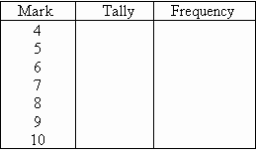Step 2:
Go through the list of marks.  The first mark in the list is 6, so put a tally mark against 6 in the second column.  The second mark in the list is 7, so put a tally mark against 7 in the second column.  The third mark in the list is 5, so put a tally mark against 5 in the third column as shown below.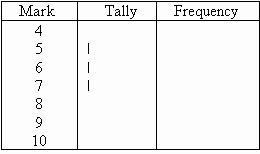We continue this process until all marks in the list are tallied.
Step 3:
Count the number of tally marks for each mark and write it in the third column.  The finished frequency table is as follows:Unlock the Problem

A frequency table is a table that uses numbers to record data about how often something happens. The frequency is the number of times the data occurs.

Example 1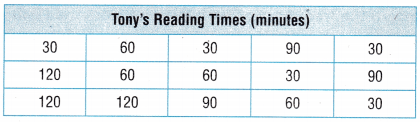Tony kept a table of the number of minutes he read during a 15 day period. He wants to represent this data in a frequency table. Make a frequency table using the data from the table.

Make a frequency table.STEP 1
List the number of minutes from Tony’s Reading Times table in the Minutes column of the frequency table.
Construct a table with three columns.  The first column shows what is being arranged in ascending order (i.e. the minutes).  The lowest minute is 30.  So, start from 30 in the first column as shown below.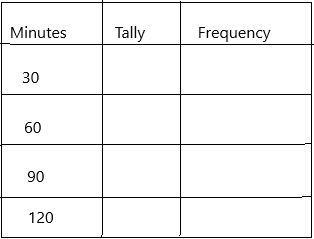STEP 2
Go through the minutes. The first minute in the list is 30, so put a tally mark against 30 in the first column.  The second minute in the list is 60, so put a tally mark against 7 in the second column.  The third minute in the list is 90, so put a tally mark against 90 in the third column as shown below. Record the frequency of the number of minutes from Tony’s Reading Table in the Frequency column.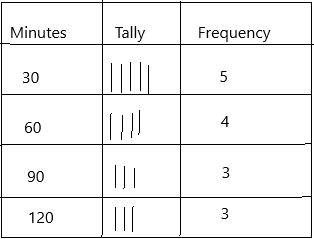Complete the frequency table.

How would the data in Tony’s table change if he recorded the number of minutes he read during a 20 day period instead of a 15 day period?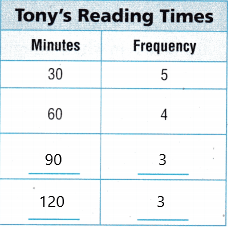Explain how the frequency table would change to show the new data Tony recorded during the 20 day period.
Answer: The minute’s column will stay the same but the frequency column will change. This will be shown below: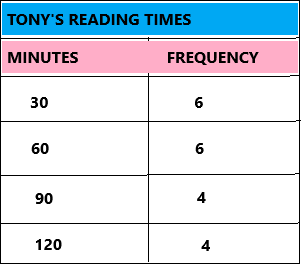Math Talk

Mathematical Processes
Explain how you used the data in Tony’s table to record the numbers in the frequency Column.
To complete the frequency column, go through the list of data values and place one tally mark at the appropriate place in the second column for every data value. Count the number of tally marks for each data value and write it in the third column.

Example 2Jasmine went for a walk each day. She recorded the distance she walked in a table. Use the data in the table to make a frequency table.

Make a frequency table to represent the data.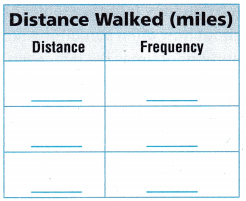STEP 1
List the distances that Jasmine walked in the Distance column of the frequency table.
Construct a table with three columns.  The first column shows what is being arranged in ascending order (i.e. the distance).  The lowest distance is 1/4.  So, start from 1/4 in the first column as shown below.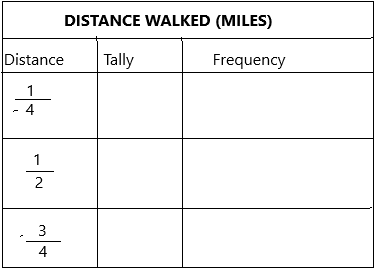STEP 2
Record the frequency of each distance from the Distance Walked table in the Frequency column.
Complete the frequency table.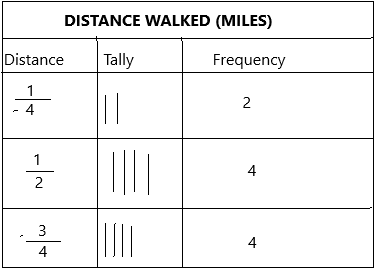Explain how creating a frequency table whose data is infractions is similar to creating a frequency table where the data is in whole numbers.
Using fractions does not change anything. because fractions are a way of expressing a division of a quantity into parts.
First, we need to know the definition of whole numbers.
Whole numbers are part of real numbers including all the positive integers and zero, but not the fractions, decimals, or negative numbers.
To convert a fraction into a whole number: Divide the numerator by the denominator, only if the numerator is a multiple of the denominator.
A fraction can be written as a whole number only if the numerator is a multiple of the denominator.
For example, convert 4/2 into a whole number.
Since 4 is a multiple of 2, we can write 4/2 = 2

Share and Show

Question 1.
Use the data on the table to complete the frequency table.STEP 1: The title of the frequency
table is __________________
The two column titles are ___________ and ____________ .
The title of the frequency table is Time Spent Doing Homework(minutes)
The two column titles are minutes and frequency.

STEP 2: List the number of minutes in the Minutes column:
________, ________, ________, ________, ________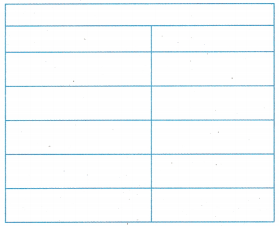The number of minutes in the minute column is:
15, 30, 45, 60, 90.
Construct a table with two columns.  The first column shows what is being arranged in ascending order (i.e. the minutes).  The lowest minute is 15.  So, start from 15 in the first column as shown below.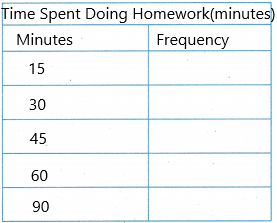STEP 3: List the frequency of the amount of time in the Frequency table:
________, ________, ________, ________, ________The frequency of the amount of time in the Frequency table:
4, 4, 3, 2, 1
Count the number for each minute and write it in the frequency column.  The finished frequency table is as follows:Question 2.
Make a frequency table using the data in the table.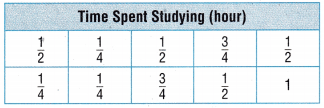Step 1:
The title of the frequency table is Time Spent Studying(hour)
The two-column titles are hour and frequency.
Step 2:
The number of hours in the hour’s column is:
1/4, 1/2, 3/4, 1.
Construct a table with two columns.  The first column shows what is being arranged in ascending order (i.e. the minutes).  The lowest hour is 1/4.  So, start from 1/4 in the first column as shown below.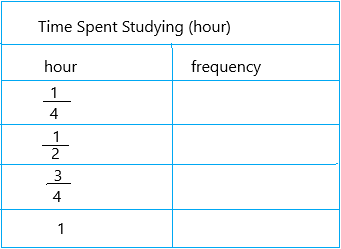Step 3:
The frequency of the amount of time in the Frequency table: 3, 4, 2, 1
Count the number for each hour and write it in the frequency column.  The finished frequency table is as follows: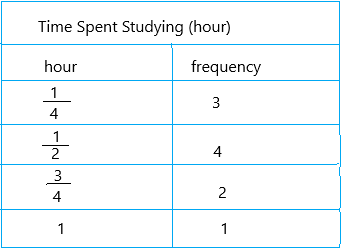Question 3.
Make a frequency table using the data in the table.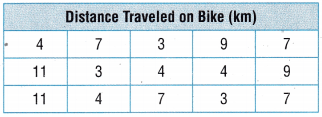Step 1:
The title of the frequency table is Distance Traveled On Bike(km)
The two-column titles are Kilometre and frequency.
Step 2:
The number of hours in the hour’s column is:
3, 4, 7, 9, 11
Construct a table with two columns. The first column shows what is being arranged in ascending order (i.e. the minutes). The lowest km is 3. So, start from 3 in the first column as shown below.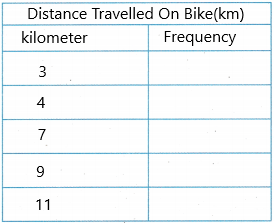Step 3:
The frequency of the amount of time in the Frequency table: 3, 4, 4, 2, 2.
Count the number for each hour and write it in the frequency column.  The finished frequency table is as follows:Problem Solving

Question 4.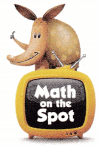H.O.T. Multi-Step Gloria likes to hike every Saturday. She records the number of miles she hikes each day. Use the data in the Distance Hiked table to make a frequency table.Step 1:
The title of the frequency table is Distance Hiked (miles).
The two-column titles are miles and frequency.
Step 2:
Write the miles in the miles column is:
7, 8, 12, 15.
Construct a table with two columns. The first column shows what is being arranged in ascending order (i.e. the miles). The lowest mile is 7. So, start from 7 in the first column as shown below.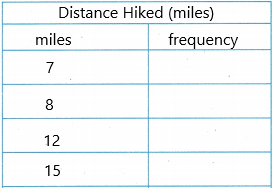Step 3:
The frequency of the amount of time in the Frequency table: 3, 6, 3, 4
Count the number for each mile and write it in the frequency column.  The finished frequency table is as follows:Question 5.
H.O.T. Explain how you would use the data in the table to make a frequency table. Then represent the data in a frequency table.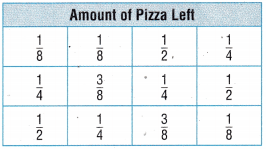Step 1:
The title of the frequency table is Amount Of Pizza Left.
The two-column titles are amount and frequency.
Step 2:
Write the amount in the Amount column is:
1/8, 1/4, 1/2, 3/8.
Construct a table with two columns. The first column shows what is being arranged in ascending order (i.e. the miles). The lowest amount is 1/8. So, start from 1/8 in the first column as shown below.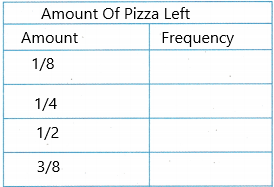Step 3:
The frequency of the amount of time in the Frequency table: 3, 4, 3, 2
Count the number for each mile and write it in the frequency column.  The finished frequency table is as follows: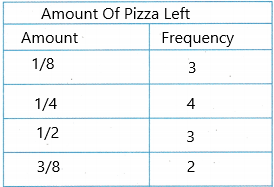Question 6.
Joe made a table to show the length of time he walked.
If Joe were to create a frequency table from this data, what number would he use to show the number of times he walked 45 minutes?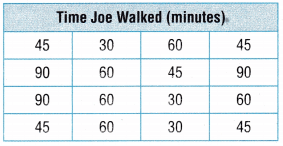(A) 2
(B) 4
(C) 3
(D) 5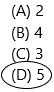Explanation:
In the table given the time Joe walked. The question asked was the number of times Joe walked 45 minutes. Count the number 45 in the table and write the frequency. In the table there are five 45’s. So the frequency is 5.Use the table at the right for 7-8.

Jennie has 4 different routes that she goes on when she walks her dog. She made a table to show when she took each of the 4 routes.

Question 7.
If Jennie was going to make a frequency table from this data, what number would she put to show the number of times she took the 1$$\frac{3}{4}$$ route?(A) 3
(B) 5
(C) 2
(D) 4Explanation:
At the table given, Jennie walked with the dog in miles. The question asked was the number would she put to show the number of times she took the 1$$\frac{3}{4}$$ route. Count the number 1$$\frac{3}{4}$$ in the table and write the frequency. In the table, there are three 1$$\frac{3}{4}$$. So the frequency is 3.Question 8.
What number would Jennie put for the frequency of $$\frac{1}{2}$$?
(A) 2
(B) 3
(C) 4
(D) 5Explanation:
Now asked the number would Jennie put for the frequency of $$\frac{1}{2}$$. Count the number would Jennie put for the frequency of $$\frac{1}{2}$$ (1/2). The frequency is 3.
Formula: $\frac{1} {a}$ = 1/a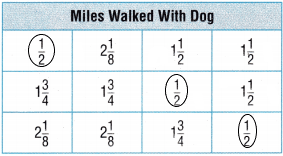TEXAS Test Prep

Question 9.
During a fund-raiser, several students were asked to sell soda during a baseball game; Danny made a table to keep track of the number of sodas students sold.

If Danny were to create a frequency table from this data, what number would he use to show the number of students who sold 10 sodas?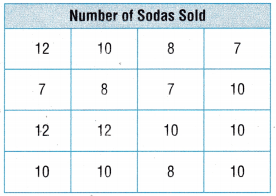(A) 3
(B) 5
(C) 10
(D) 7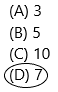Explanation:
In the table given the number of sodas Danny sold. The question asked was the number would he use to show the number of students who sold 10 sodas. Count the number 10’s in the table and write the frequency. In the table there are seven 10’s. So the frequency is 7.### Texas Go Math Grade 4 Lesson 17.1 Homework and Practice Answer Key

Question 1.
Make a frequency table using the data in the table.Step 1:
The title of the frequency table is Books Checked Out
The two-column titles are the Number of books and frequency.
Step 2:
The number of books in the books column is:
2, 3, 5, 6, 7.
Construct a table with two columns.  The first column shows what is being arranged in ascending order (i.e. the books).  The lowest hour is 2.  So, start from 2 in the first column as shown below.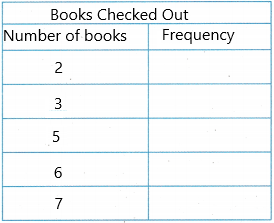Step 3:
The frequency of the number of books in the Frequency table: 4, 5, 2, 3, 1
Count the number for each book and write it in the frequency column.  The finished frequency table is as follows:Question 2.
Make a frequency table using the data in the table.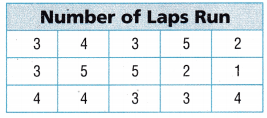Step 1:
The title of the frequency table is Number Of Laps Run.
The two-column titles are the Number and frequency.
Step 2:
The number of books in the books column is:
1, 2, 3, 4, 5.
Construct a table with two columns.  The first column shows what is being arranged in ascending order (i.e. the number).  The lowest number is 1.  So, start from 1 in the first column as shown below.Step 3:
The frequency of the number of laps run in the Frequency table: 1, 2, 5, 4, 3.
Count the number for each lap run and write it in the frequency column.  The finished frequency table is as follows:Problem Solving

Question 3.
Paul made a table to show how many hits each baseball player got in 2 games. Use the data from the table to make a frequency table.Step 1:
The title of the frequency table is Number Of Hits.
The two-column titles are the Number and frequency.
Step 2:
The number of hits in the number column is:
0,1, 2, 3, 4.
Construct a table with two columns.  The first column shows what is being arranged in ascending order (i.e. the number).  The lowest number is 0.  So, start from 0 in the first column as shown below.Step 3:
The frequency of the number of hits in the Frequency table: 3, 6, 4, 3, 2.
Count the number for each hit and write it in the frequency column.  The finished frequency table is as follows:Question 4.
Sahara made a table to show how many cans were left in the recycle bin each day. Use the data from the table to make a frequency table.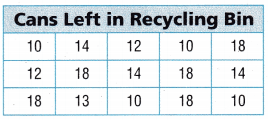Step 1:
The title of the frequency table is Cans Left in Recycling Bin.
The two-column titles are the number and frequency.
Step 2:
The number of cans in the number column is:
10, 12, 13, 14, 18.
Construct a table with two columns.  The first column shows what is being arranged in ascending order (i.e. the number).  The lowest number is 10.  So, start from 10 in the first column as shown below.Step 3:
The frequency of the number of cans left in the Frequency table: 4, 2, 1, 3, 5.
Count the number for each can and write it in the frequency column.  The finished frequency table is as follows: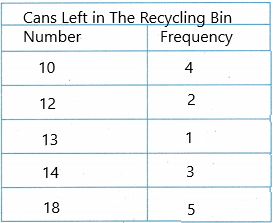Lesson Check

Question 5.If Greg were to make a frequency table from this data, what number would he use to show the number of times he made 14 baskets?
(A) 5
(B) 0
(C) 3
(D) 4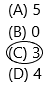Explanation:
In the table given the number of baskets made. The question asked was the number would he use to show the number of times he made 14 baskets. Count the number 14’s in the table and write the frequency. In the table there are three 14’s. So the frequency is 3.Question 6.
Lacy made a table to show the number of miles she walked.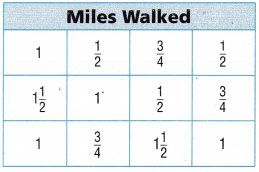If Lacy were to make a frequency table from this data, what number would she use to show the number of times she walked $$\frac{1}{2}$$ mile?
(A) 4
(B) 2
(C) 1
(D) 3Explanation:
Now asked the number would Lacy put for the frequency of $$\frac{1}{2}$$. Count the number would Jennie put for the frequency of $$\frac{1}{2}$$ (1/2). The frequency is 3.
Formula: $\frac{1} {a}$ = 1/aUse the table at right for 7 – 8.

Ellen is training to run a marathon. She made a table to show how many miles she runs each day she trains.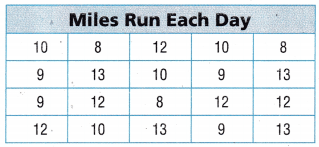Question 7.
Multi-Step Ellen made a frequency table from this data. Which mileage will have the greatest frequency?
(A) 12
(B) 13
(C) 9
(D) 10Explanation:
Ellen already made a frequency table. Here asked which mileage has a higher frequency. We need to count each mileage and circle the mileage which has the highest.Question 8.
Multi-Step Which statement about the frequency table Ellen made is NOT true?
(A) Ellen ran the same number of 9 and 10 mile days.
(B) Ellen ran more 12 mile days than 10 mile days.
(C) Ellen ran four 9, 10, and 13 mile days.
(D) Ellen ran fewer 9 mile days than 8 mile days.